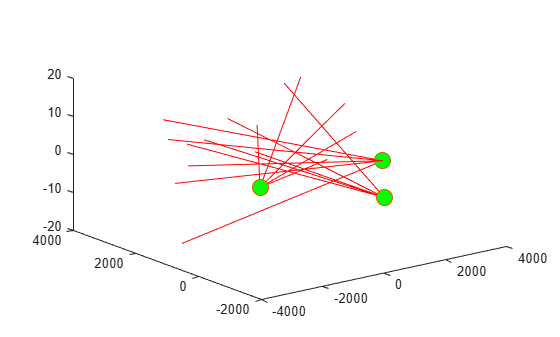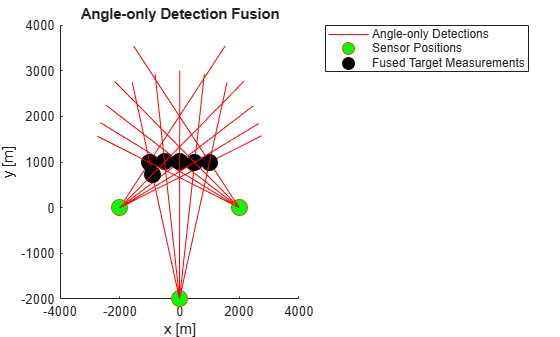staticDetectionFuser

Static fusion of synchronous sensor detections

Description

staticDetectionFuser System object™ creates a static detection fuser object to fuse angle-only sensor detections.

To obtain the fuser:

1. Create the staticDetectionFuser object and set its properties.

2. Call the object with arguments, as if it were a function.

Creation

Description

fuser = staticDetectionFuser() creates a default three-sensor static detection fuser object to fuse angle-only sensor detections.

example

fuser = staticDetectionFuser(Name,Value) sets properties using one or more name-value pairs. For example, fuser = staticDetectionFuser('FalseAlarmRate',1e-6,'MaxNumSensors',12) creates a fuser that has a maximum of 12 sensors and a false alarm rate of 1e-6. Enclose each property name in quotes.

Properties

expand all

Unless otherwise indicated, properties are nontunable, which means you cannot change their values after calling the object. Objects lock when you call them, and the release function unlocks them.

If a property is tunable, you can change its value at any time.

Sensor index of the composite detections reported by the fuser, specified as a positive integer. This index becomes the SensorIndex of objectDetection objects returned by the fuser.

Example: 5

Data Types: double

Function for fusing multiple sensor detections, specified as a character vector, string, or function handle. The function fuses multiple detections into one and returns the fused measurement and measurement noise. Any fusing function combines at most one detection from each sensor. The syntax of the measurement fuser function is:

[fusedMeasurement,fusedMeasurementNoise] = MeasurementFusionFcn(detections)
where the input and output functions arguments are

• detections – cell array of objectDetection measurements.

• fusedMeasurement – an N-by-1 vector of fused measurements.

• fusedMeasurementNoise – an N-by-N matrix of fused measurements noise.

The value of N depends on the MeasurementFormat property.

 MeasurementFormat Property N 'Position' 1, 2, and 3 'Velocity 1, 2, and 3 'PositionAndVelocity 2, 4, and 6 'Custom' Any

Data Types: char | string | function_handle

Format of the fused measurement, specified as 'Position', 'Velocity', 'PositionAndVelocity', or 'Custom'. The formats are

• 'Position' – the fused measurement is the position of the target in the global coordinate frame.

• 'Velocity' – the fused measurement is the velocity of the target in the global coordinate frame.

• 'PositionAndVelocity' – the fused measurement is the position and velocity of the target in the global coordinate frame defined according to the format [x;vx;y;vy;z;vz].

• 'Custom' – custom fused measurement. To enable this format, specify a function using the MeasurementFcn.

Example: 'PositionAndVelocity'

Custom measurement function, specified as a character vector, string, or function handle. Specify the function that transforms fused measurements into sensor measurements. The function must have the following signature:

sensorMeas = MeasurementFcn(fusedMeas,measParameters)

Dependencies

To enable this property, set the MeasurementFormat property to 'Custom'.

Data Types: char | string | function_handle

Maximum number of sensors in surveillance region, specified as a positive integer greater than one.

Data Types: double

Volume of sensors detection bins, specified as a positive scalar or N-length vector of positive scalars. N is the number of sensors. If specified as a scalar, each sensor is assigned the same volume. If a sensor produces an angle-only measurement, for example, azimuth and elevation, the volume is defined as the solid angle subtended by one bin.

Data Types: double

Probability of detection of a target by each sensor, specified as a scalar or N-length vector of positive scalars in the range (0,1). N is the number of sensors. If specified as a scalar, each sensor is assigned the same detection probability. The probability of detection is used in calculating the cost of fusing a "one" (target was detected) or "zero" (target was not detected) detections from each sensor.

Example: 0.99

Data Types: double

Rate at which false positives are reported by sensor in each bin, specified as a scalar or N-length vector of positive scalars. N is the number of sensors. If specified as a scalar, each sensor is assigned the same false alarm rate. The false alarm rate is used to calculate the likelihood of clutter in the detections reported by each sensor.

Example: 1e-5

Data Types: double

Option to use parallel computing resources, specified as false or true. The staticDetectionFuser calculates the cost of fusing detections from each sensor as an n-D assignment problem. The fuser spends most of the time in computing the cost matrix for the assignment problem. If Parallel Computing Toolbox™ is installed, this option lets the fuser use the parallel pool of workers to compute the cost matrix.

Data Types: logical

Absolute tolerance between timestamps of detections, specified as a nonnegative scalar. The staticDetectionFuser assumes that sensors are synchronous. This property defines the allowed tolerance value between detection time-stamps to still be considered synchronous.

Example: 1e-3

Data Types: double

Usage

Description

compositeDets = fuser(dets) returns the fused detections, compositeDets, of input detections, dets.

[compositeDets,analysisInfo] = fuser(dets) also returns analysis information, analysisInfo.

Input Arguments

expand all

Pre-fused detections, specified as a cell array of objectDetection objects.

Output Arguments

expand all

Pre-fused detections, returned as a cell array of objectDetection objects.

Analysis information, returned as a structure. The fields of the structure are:

• CostMatrixN-dimensional cost matrix providing the cost of association of detections, where N is the number of sensors. The cost is the negative log-likelihood of the association and can be interpreted as the negative score of the track that will be generated by the fused measurement.

• Assignments – A P-by-N list of assignments, where P is the number of composite detections.

• FalseAlarms – A Q-by-1 list of indices of detections declared as false alarms by association.

Data Types: struct

Object Functions

To use an object function, specify the System object as the first input argument. For example, to release system resources of a System object named obj, use this syntax:

release(obj)

expand all

 release Release resources and allow changes to System object property values and input characteristics reset Reset internal states of System object isLocked Determine if System object is in use clone Create duplicate System object

Examples

collapse all

Fuse angle-only detections from three ESM sensors.

Load stored detections from the sensors.

Visualize angle-only detections for plotting the direction vector.

rPlot = 5000;
plotData = zeros(3,numel(detections)*3);
for i = 1:numel(detections)
az = detections{i}.Measurement(1);
el = detections{i}.Measurement(2);
% The sensor is co-located at platform center, therefore use
% the position from the second measurement parameter
originPos = detections{i}.MeasurementParameters(2).OriginPosition;
positionData(:,i) = originPos(:);
plotData(:,3*i-2) = [xt;yt;zt] + originPos(:);
plotData(:,3*i-1) = originPos(:);
plotData(:,3*i) = [NaN;NaN;NaN];
end
plot3(plotData(1,:),plotData(2,:),plotData(3,:),'r-')
hold on
plot3(positionData(1,:),positionData(2,:),positionData(3,:),'o','MarkerSize',12,'MarkerFaceColor','g')Create a staticDetectionFuser to fuse angle-only detections using the measurement fusion function triangulateLOS.

fuser = staticDetectionFuser('MeasurementFusionFcn','triangulateLOS','MaxNumSensors',3)
fuser =
staticDetectionFuser with properties:

FusedSensorIndex: 1
MeasurementFusionFcn: 'triangulateLOS'
MeasurementFormat: 'Position'

MaxNumSensors: 3
Volume: [3x1 double]
DetectionProbability: [3x1 double]
FalseAlarmRate: [3x1 double]

TimeTolerance: 1.0000e-06
UseParallel: false

Create the fused detections and obtain the analysis information.

[fusedDetections, analysisInfo] = fuser(detections);
fusedPositions = zeros(3,numel(fusedDetections));
for i = 1:numel(fusedDetections)
fusedPositions(:,i) = fusedDetections{i}.Measurement;
end
plot3(fusedPositions(1,:),fusedPositions(2,:),fusedPositions(3,:),'ko', ...
'MarkerSize',12, 'MarkerFaceColor','k')
legend('Angle-only Detections','Sensor Positions','Fused Target Measurements')
title('Angle-only Detection Fusion')
xlabel('x [m]')
ylabel('y [m]')
view(2)Use the analysisInfo output to check the assignments.

analysisInfo.Assignments
ans = 6x3 uint32 matrix

0   10   14
1    6   11
2    7   12
3    8   13
4    9    0
5    0   15

Algorithms

expand all

 Bar-Shalom, Yaakov, Peter K. Willett, and Xin Tian. Tracking and data fusion. Storrs, CT, USA:: YBS publishing, 2011.